UP PREV NEXT

### 9. Summary of method and results

Initial circuit Timing overview Best stage effort Library mapping Gate retiming Input buffering Better accuracy Prior art Summary Conclusions

#### Problem statement

The problem is to size the gates of a 4-bit adder shown on the right to meet a certain timing, input capacitance and output load specification. U.S. Patent number 6,725,438 describes a first method for the gate sizing using a single area coefficient. The second method uses two area coefficients. The third method using the prior art sizes the gates using the input pin capacitance.

The methods are tested using the vsclib characterised using a generic 0.13um technology.

#### Single area coefficient algorithm

The patent describes a method for analysing the cells in a standard cell library and deriving a coefficient CS which allows the delay of a cell to be expressed as a function of its load and area.

The stage effort of each gate is set to 3.6 to produce an initial circuit timing. This is then adjusted for each critical path in turn. Gates on paths with positive slack have their stage effort reduced by an equal amount. Gates on paths with negative slack have their stage effort increased by an equal amount until all paths have zero slack.

Starting from the output, and given each gate's delay, their input capacitance can be calculated using expressions from logical effort theory. The input capacitance plus any wireload capacitance is the output capacitance of previous gates.

The area of each cell can be estimated from its delay using the coefficient CS and its load COUT. This is used to estimate the total circuit area and to select the cell with the nearest area from the library.

Fig 9a. Initial 4-bit adder circuit to meet timing of 350ps driving 35fF with input capacitance of 35fF.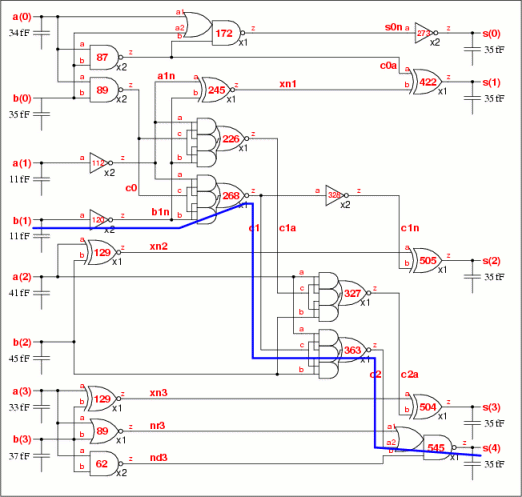stage effort f = gCOUT /CIN

gate delay d = τ ( p+f )

input capacitance CIN = gCOUT /( d/τ - p )

Definitions are on the Overview of CMOS timing models page.

cell area A = COUT /(CS × ( d/τ - p))

#### Two area coefficients algorithm

Two area coefficients CS0 and CS1 to estimate the area from the delay give a better estimate than a single CS one.

cell area A = {COUT /( d/τ -p)-CS0 }/CS1

#### Prior art

The book Logical Effort, Designing Fast CMOS Circuits by Ivan Sutherland, Bob Sproull and David Harris published by Morgan Kaufmann Publishers in 1999 gives the basic logical effort theory and equations.

Each path is analysed and its total effort delay found by subtracting the parasitic delay from the path delay. Then the stage effort of each gate is the total effort delay divided by the number of stages. The critical path is the one with the lowest stage effort.

The critical paths are evaluated in turn and used to assign stage efforts to each gate. Then working backwards from the outputs, each gate's input capacitance is calculated using the stage effort.

The standard cell with the next highest input capacitance is chosen from the library.

effort delay DF = D-P

stage effort = DF /N

input capacitance CIN = gCOUT /f

#### Initial schematic for the patent algorithm and the prior art

The schematic Fig 9b on the left below shows the fixed timing from a stage effort of 3.6 for the 4-bit adder. The schematic Fig 9c on the right below shows the stage efforts of each gate calculated according to the logical effort theory.

Fig 9b. 4-bit adder fixed delays.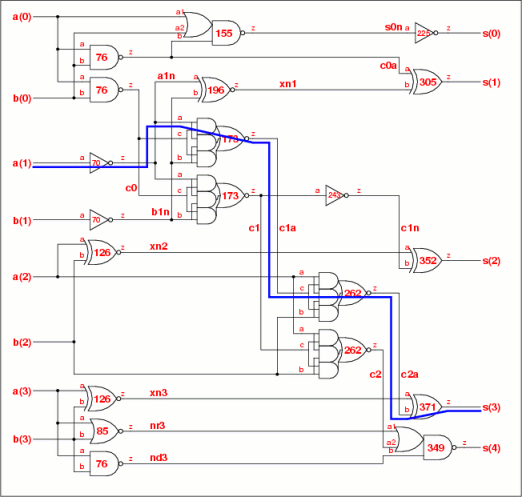Fig 9c. 4-bit adder stage efforts.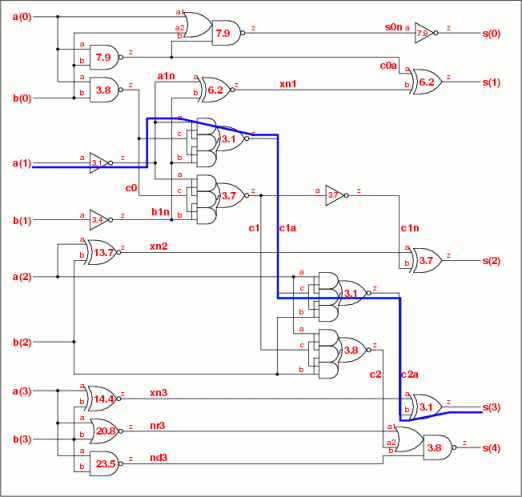#### Comprison of patent algorithm to prior art

The patent algorithm actually does the same operations to the circuit as the prior art. As a result the final netlist is nearly the same.

What is new in the patent is

1. The use of an arbitrary stage effort for initial timing and then adjusting it by compressing or stretching delays, rather than calculating each cell's stage effort by directly using the path parasitic delay.
2. Linking area to delay by a single area coefficient so that area estimates can be made without using lookup tables to the library information.
3. Using the area estimate of each cell instead of the input capacitance to select the cells from the library.

#### Design considerations

The method described in the patent does not by itself lead to the right solution for the 4-bit adder optimisation exercise.
1. Non-inverting cells do not fit well (eg see the Choosing the best stage effort page) the fixed parasitic delay and logical effort model of logical effort. Neither the patent nor the prior art explain how to overcome this limitation. In order to limit the errors, the maximum gain of non-inverting gates has been set to 5.
2. The patent algorithm chooses the nearest cell by area and can choose a cell which is too weak, causing the path delay to be above the spec value. The prior art algorithm uses the estimated input capacitance to choose the next cell up. If the same is done with the estimated area in the patent algorithm, then the area estimates will be too low.
3. Buffer insertion is described in the patent, but exclusively from the perspective of reducing area. Buffer insertion to improve timing, needed for the 4-bit adder solution, is not covered.
Given the above, the buffered 4-bit adder schematics produced by the patent algorithm and by the prior art are shown below.
Fig 9d. Buffered 4-bit adder using patent algorithm with a single area coefficient CS.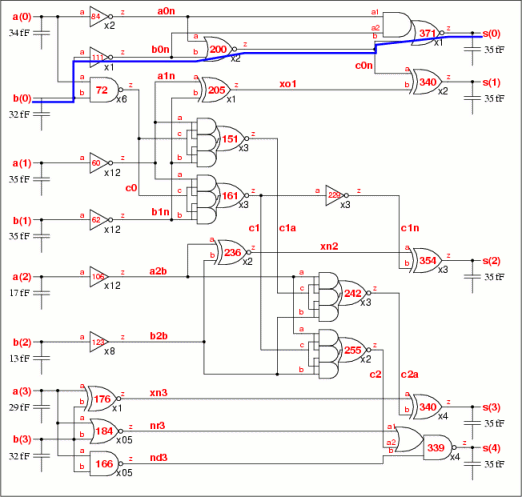86.7 gates
Fig 9e. Buffered 4-bit adder using prior art.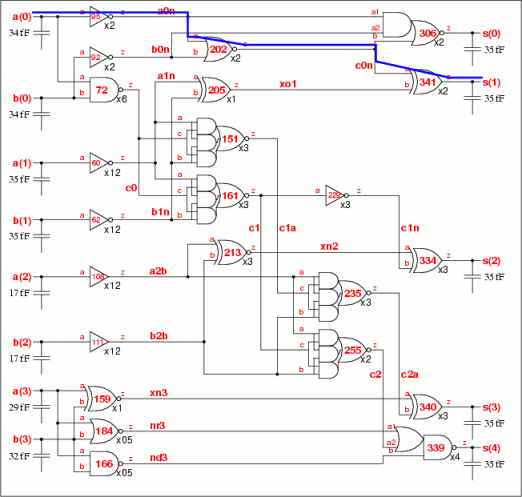89.3 gates
The schematic produced by the patent algorithm fails to meet the timing of 350ps. The timing fails also when using two area coefficients for mapping the library cells (Fig 7c). The problem is the undersized instances b0n and s0. The schematic produced by the prior art algorithm meets timing.
 UP PREV NEXT 6-AUG-05